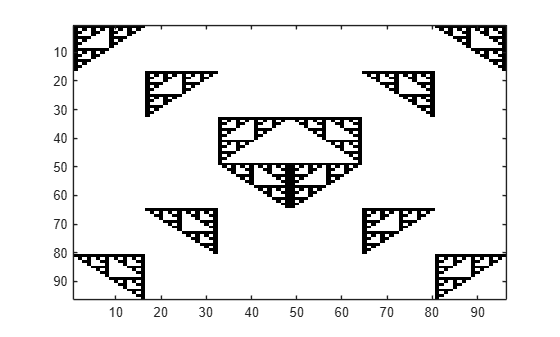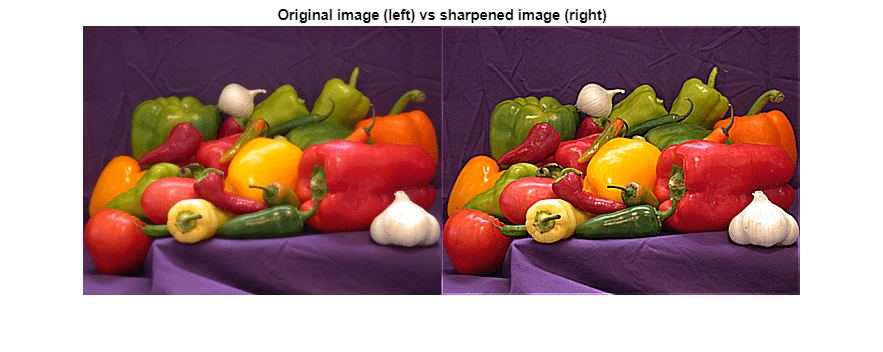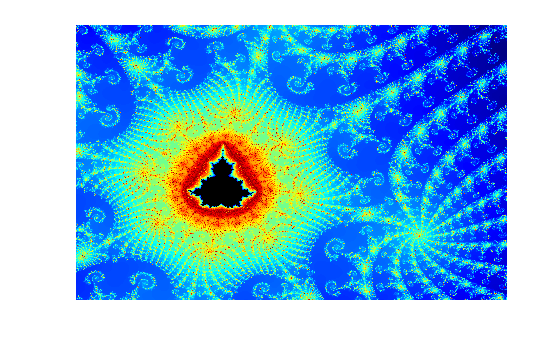Run MATLAB Functions on a GPU

MATLAB Functions with gpuArray Arguments

Hundreds of functions in MATLAB® and other toolboxes run automatically on a GPU if you supply a gpuArray argument.

A = gpuArray([1 0 1; -1 -2 0; 0 1 -1]);
e = eig(A);

Whenever you call any of these functions with at least one gpuArray as a data input argument, the function executes on the GPU. The function generates a gpuArray as the result, unless returning MATLAB data is more appropriate (for example, size). You can mix inputs using both gpuArray and MATLAB arrays in the same function call. To learn more about when a function runs on GPU or CPU, see Special Conditions for gpuArray Inputs. gpuArray-enabled functions include the discrete Fourier transform (fft), matrix multiplication (mtimes), left matrix division (mldivide), and hundreds of others. For more information, see Check gpuArray-Supported Functions.

Check gpuArray-Supported Functions

If a MATLAB function has support for gpuArray objects, you can consult additional GPU usage information on its function page. See GPU Arrays in the Extended Capabilities section at the end of the function page.

Tip

For a filtered list of MATLAB functions that support gpuArray objects, see Function List (GPU-arrays).

Several MATLAB toolboxes include functions with built-in gpuArray support. To view lists of all functions in these toolboxes that support gpuArray objects, use the links in the following table. Functions in the lists with information indicators have limitations or usage notes specific to running the function on a GPU. You can check the usage notes and limitations in the Extended Capabilities section of the function reference page. For information about updates to individual gpuArray-enabled functions, see the release notes.

Toolbox NameList of Functions with gpuArray SupportGPU-Specific Documentation
MATLABFunctions with gpuArray support
Statistics and Machine Learning Toolbox™Functions with gpuArray support (Statistics and Machine Learning Toolbox)Analyze and Model Data on GPU (Statistics and Machine Learning Toolbox)
Image Processing Toolbox™Functions with gpuArray support (Image Processing Toolbox)GPU Computing (Image Processing Toolbox)
Deep Learning Toolbox™

Functions with gpuArray support (Deep Learning Toolbox)

Scale Up Deep Learning in Parallel, on GPUs, and in the Cloud (Deep Learning Toolbox)

Deep Learning with MATLAB on Multiple GPUs (Deep Learning Toolbox)

Computer Vision Toolbox™Functions with gpuArray support (Computer Vision Toolbox)GPU Code Generation and Acceleration (Computer Vision Toolbox)
Communications Toolbox™Functions with gpuArray support (Communications Toolbox)Code Generation and Acceleration Support (Communications Toolbox)
Signal Processing Toolbox™Functions with gpuArray support (Signal Processing Toolbox)Code Generation and GPU Support (Signal Processing Toolbox)
Audio Toolbox™Functions with gpuArray support (Audio Toolbox)Code Generation and GPU Support (Audio Toolbox)
Wavelet Toolbox™Functions with gpuArray support (Wavelet Toolbox)Code Generation and GPU Support (Wavelet Toolbox)
Curve Fitting Toolbox™Functions with gpuArray support (Curve Fitting Toolbox)

You can browse gpuArray-supported functions from all MathWorks® products at the following link: gpuArray-supported functions. Alternatively, you can filter by product. On the Help bar, click Functions. In the function list, browse the left pane to select a product, for example, MATLAB. At the bottom of the left pane, select GPU Arrays. If you select a product that does not have gpuArray-enabled functions, then the GPU Arrays filter is not available.

Deep Learning with GPUs

For many functions in Deep Learning Toolbox, GPU support is automatic if you have a suitable GPU and Parallel Computing Toolbox™. You do not need to convert your data to gpuArray. The following is a non-exhaustive list of functions that, by default, run on the GPU if available.

For more information about automatic GPU support in Deep Learning Toolbox, see Scale Up Deep Learning in Parallel, on GPUs, and in the Cloud (Deep Learning Toolbox).

For advanced networks and workflows that use networks defined as dlnetwork (Deep Learning Toolbox) objects or model functions, convert your data to gpuArray. Use functions with gpuArray support (Deep Learning Toolbox) to run custom training loops or prediction on the GPU.

Check or Select a GPU

If you have a GPU, then MATLAB automatically uses it for GPU computations. You can check and select your GPU using the gpuDevice function. If you have multiple GPUs, then you can use gpuDeviceTable to examine the properties of all GPUs detected in your system. You can use gpuDevice to select one of them, or use multiple GPUs with a parallel pool. For an example, see Identify and Select a GPU Device and Use Multiple GPUs in Parallel Pool. To check if your GPU is supported, see GPU Support by Release.

For deep learning, MATLAB provides automatic parallel support for multiple GPUs. See Deep Learning with MATLAB on Multiple GPUs (Deep Learning Toolbox).

Use MATLAB Functions with the GPU

This example shows how to use gpuArray-enabled MATLAB functions to operate with gpuArray objects. You can check the properties of your GPU using the gpuDevice function.

gpuDevice
ans =

Name: 'TITAN RTX'
Index: 1
ComputeCapability: '7.5'
SupportsDouble: 1
DriverVersion: 11.2000
ToolkitVersion: 11
MaxShmemPerBlock: 49152
MaxGridSize: [2.1475e+09 65535 65535]
SIMDWidth: 32
TotalMemory: 2.5770e+10
AvailableMemory: 2.4177e+10
MultiprocessorCount: 72
ClockRateKHz: 1770000
ComputeMode: 'Default'
GPUOverlapsTransfers: 1
KernelExecutionTimeout: 1
CanMapHostMemory: 1
DeviceSupported: 1
DeviceAvailable: 1
DeviceSelected: 1

Create a row vector that repeats values from -15 to 15. To transfer it to the GPU and create a gpuArray object, use the gpuArray function.

X = [-15:15 0 -15:15 0 -15:15];
gpuX = gpuArray(X);
whos gpuX
Name      Size            Bytes  Class       Attributes

gpuX      1x95              760  gpuArray

To operate with gpuArray objects, use any gpuArray-enabled MATLAB function. MATLAB automatically runs calculations on the GPU. For more information, see Run MATLAB Functions on a GPU. For example, use diag, expm, mod, round, abs, and fliplr together.

gpuE = expm(diag(gpuX,-1)) * expm(diag(gpuX,1));
gpuM = mod(round(abs(gpuE)),2);
gpuF = gpuM + fliplr(gpuM);

Plot the results.

imagesc(gpuF);
colormap(flip(gray));If you need to transfer the data back from the GPU, use gather. Transferring data back to the CPU can be costly, and is generally not necessary unless you need to use your result with functions that do not support gpuArray.

result = gather(gpuF);
whos result
Name         Size            Bytes  Class     Attributes

result      96x96            73728  double

In general, running code on the CPU and the GPU can produce different results due to numerical precision and algorithmic differences between the GPU and CPU. Answers from the CPU and GPU are both equally valid floating point approximations to the true analytical result, having been subjected to different roundoff behavior during computation. In this example, the results are integers and round eliminates the roundoff errors.

Sharpen an Image Using the GPU

This example shows how to sharpen an image using gpuArrays and GPU-enabled functions.

Read the image, and send it to the GPU using the gpuArray function.

Convert the image to doubles, and apply convolutions to obtain the gradient image. Then, using the gradient image, sharpen the image by a factor of amount.

dimage = im2double(image);
amount = 5;

Resize, plot and compare the original and sharpened images.

imshow(imresize([dimage, sharpened],0.7));
title('Original image (left) vs sharpened image (right)');Compute the Mandelbrot Set using GPU-Enabled Functions

This example shows how to use GPU-enabled MATLAB functions to compute a well-known mathematical construction: the Mandelbrot set. Check your GPU using the gpuDevice function.

Define the parameters. The Mandelbrot algorithm iterates over a grid of real and imaginary parts. The following code defines the number of iterations, grid size, and grid limits.

maxIterations = 500;
gridSize = 1000;
xlim = [-0.748766713922161, -0.748766707771757];
ylim = [ 0.123640844894862,  0.123640851045266];

You can use the gpuArray function to transfer data to the GPU and create a gpuArray, or you can create an array directly on the GPU. gpuArray provides GPU versions of many functions that you can use to create data arrays, such as linspace. For more information, see Create GPU Arrays Directly.

x = gpuArray.linspace(xlim(1),xlim(2),gridSize);
y = gpuArray.linspace(ylim(1),ylim(2),gridSize);
whos x y
Name      Size              Bytes  Class       Attributes

x         1x1000             8000  gpuArray
y         1x1000             8000  gpuArray

Many MATLAB functions support gpuArrays. When you supply a gpuArray argument to any GPU-enabled function, the function runs automatically on the GPU. For more information, see Run MATLAB Functions on a GPU. Create a complex grid for the algorithm, and create the array count for the results. To create this array directly on the GPU, use the ones function, and specify 'gpuArray'.

[xGrid,yGrid] = meshgrid(x,y);
z0 = complex(xGrid,yGrid);
count = ones(size(z0),'gpuArray');

The following code implements the Mandelbrot algorithm using GPU-enabled functions. Because the code uses gpuArrays, the calculations happen on the GPU.

z = z0;
for n = 0:maxIterations
z = z.*z + z0;
inside = abs(z) <= 2;
count = count + inside;
end
count = log(count);

When computations are done, plot the results.

imagesc(x,y,count)
colormap([jet();flipud(jet());0 0 0]);
axis offWork with Sparse Arrays on a GPU

The following functions support sparse gpuArray objects.

 abs acos acosd acosh acot acotd acoth acsc acscd acsch angle asec asecd asech asin asind asinh atan atand atanh bicg bicgstab ceil cgs classUnderlying conj cos cosd cosh cospi cot cotd coth csc cscd csch ctranspose deg2rad diag end eps exp expint expm1 find fix floor full gmres gpuArray.speye imag isaUnderlying isdiag isempty isequal isequaln isfinite isfloat isinteger islogical isnumeric isreal issparse istril istriu isUnderlyingType length log log2 log10 log1p lsqr minus mtimes mustBeUnderlyingType ndims nextpow2 nnz nonzeros norm numel nzmax pcg plus qmr rad2deg real reallog realsqrt round sec secd sech sign sin sind sinh sinpi size sparse spfun spones sprandsym sqrt sum tan tand tanh tfqmr times (.*) trace transpose tril triu uminus underlyingType uplus

You can create a sparse gpuArray either by calling sparse with a gpuArray input, or by calling gpuArray with a sparse input. For example,

x = [0 1 0 0 0; 0 0 0 0 1]
0     1     0     0     0
0     0     0     0     1
s = sparse(x)
(1,2)        1
(2,5)        1
g = gpuArray(s);   % g is a sparse gpuArray
gt = transpose(g); % gt is a sparse gpuArray
f = full(gt)       % f is a full gpuArray
0     0
1     0
0     0
0     0
0     1

Sparse gpuArray objects do not support indexing. Instead, use find to locate nonzero elements of the array and their row and column indices. Then, replace the values you want and construct a new sparse gpuArray.

Work with Complex Numbers on a GPU

If the output of a function running on the GPU could potentially be complex, you must explicitly specify its input arguments as complex. This applies to gpuArray or to functions called in code run by arrayfun.

For example, if creating a gpuArray that might have negative elements, use G = gpuArray(complex(p)), then you can successfully execute sqrt(G).

Or, within a function passed to arrayfun, if x is a vector of real numbers, and some elements have negative values, sqrt(x) generates an error; instead you should call sqrt(complex(x)).

If the result is a gpuArray of complex data and all the imaginary parts are zero, these parts are retained and the data remains complex. This could have an impact when using sort, isreal, and so on.

The following table lists the functions that might return complex data, along with the input range over which the output remains real.

FunctionInput Range for Real Output
acos(x)abs(x) <= 1
acosh(x)x >= 1
acoth(x)abs(x) >= 1
acsc(x)abs(x) >= 1
asec(x)abs(x) >= 1
asech(x)0 <= x <= 1
asin(x)abs(x) <= 1
atanh(x)abs(x) <= 1
log(x)x >= 0
log1p(x)x >= -1
log10(x)x >= 0
log2(x)x >= 0
power(x,y)x >= 0
reallog(x)x >= 0
realsqrt(x)x >= 0
sqrt(x)x >= 0

Special Conditions for gpuArray Inputs

GPU-enabled functions run on the GPU only when the data is on the GPU. For example, the following code runs on GPU because the data, the first input, is on the GPU:

>> sum(gpuArray(magic(10)),2);
However, this code does not run on GPU because the data, the first input, is not on the GPU:
>> sum(magic(10),gpuArray(2));
If your input argument gpuArray objects contain items such as dimensions, scaling factors, or number of iterations, then the function gathers them and computes on the CPU. Functions only run on the GPU when the actual data arguments are gpuArray objects.

Acknowledgments

MAGMA is a library of linear algebra routines that take advantage of GPU acceleration. Linear algebra functions implemented for gpuArray objects in Parallel Computing Toolbox leverage MAGMA to achieve high performance and accuracy.